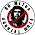90 Miles From Tyranny : A Simple Logic Question That Most Harvard Students Get Wrong

## Friday, March 11, 2016

### A Simple Logic Question That Most Harvard Students Get Wrong

Harvard students get near-perfect SAT scores. These are smart, smart kids. So they shouldn't have trouble with a simple logic question, right?

Try the following puzzle:

A bat and ball cost \$1.10.

The bat costs one dollar more than the ball.

How much does the ball cost?

Scroll down for the answer ...

But the real answer is 5¢.

Behavioral economist Daniel Kahneman explains why most people get this wrong:

A number came to your mind. The number, of course, is 10: 10¢. The distinctive mark of this easy puzzle is that it evokes an answer that is intuitive, appealing, and wrong. Do the math, and you will see. If the ball costs 10¢, then the total cost will be \$1.20 (10¢ for the ball and \$1.10 for the bat), not \$1.10. The correct answer is 5¢. It is safe to assume that the intuitive answer also came to the mind of those who ended up with the correct number—they somehow managed to resist the intuition.

Many thousands of university students have answered the bat-and-ball puzzle, and the results are shocking. More than 50% of students at Harvard, MIT, and Princeton gave the intuitive—incorrect—answer. At less selective universities, the rate of demonstrable failure to check was in excess of 80%. The bat-and-ball problem is our first encounter with an observation that will be a recurrent theme of this book: many people are overconfident, prone to place too much faith in their intuitions. They apparently find cognitive effort at least mildly unpleasant and avoid it as much as possible.

This excerpt comes from Kahneman's 2011 book, "Thinking, Fast And Slow," which posits that we have an intuitive mental system and a logical mental system, and we often use the wrong one at the wrong time.

More Logic:

### The Modus Operandi Of The Left...

Source

1.I'm gonna borrow one of these...giving you full credit of course.

1.Sounds good to me!

2.Not a logic puzzle. It's a basic math problem. Two equations with two unknowns.
1: Ball + Bat = 1.10
2: Bat - 1 = Ball.
-------------------
Substitute 2 into 1: (bat - 1) + Bat = 1.10
Simplify: 2Bat - 1 = 1.10
2Bat = 2.10
Bat = 1.05
Solve for Ball, given Bat = 1.05: Ball = .05